International
Tables for
Crystallography
Volume C
Mathematical, physical and chemical tables
Edited by E. Prince

International Tables for Crystallography (2006). Vol. C, ch. 6.2, p. 596

## Section 6.2.1. Expressions for intensity of diffraction

H. Lipson,a J. I. Langforda and H.-C. Hub

aSchool of Physics & Astronomy, University of Birmingham, Birmingham B15 2TT, England, and bChina Institute of Atomic Energy, PO Box 275 (18), Beijing 102413, People's Republic of China

### 6.2.1. Expressions for intensity of diffraction

| top | pdf |

The expressions for the intensity of diffraction of X-rays contain several trigonometrical factors. The earlier series of International Tables (Kasper & Lonsdale, 1959, 1972) gave extensive tables of these functions, but such tables are now unnecessary, as the functions are easily computed. In fact, many crystallographers can ignore the trigonometric factors entirely, as they are built into `black-box' data-processing programs. The formulae for single-crystal reflections (b) and (c) of Table 6.2.1.1in the previous edition (Lipson & Langford, 1998) list only the integrated reflection power ratio (i.e. integrated reflection) under the strong absorption case. The revised formulae given here include both the reflection power ratio and the integrated reflection power ratio for a crystal slab of finite thickness with any values of the ratio of the absorption to the diffraction cross sections and under all possible kinds of diffraction geometry.

 Table 6.2.1.1| top | pdf | Summary of formulae for integrated powers of reflection
 Absorption is neglected in both (g) and (h).
 (a) Crystal element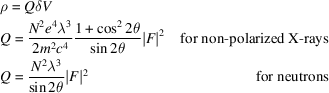(b) Reflection from a crystal slab of thickness t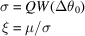1. Symmetrical Bragg geometry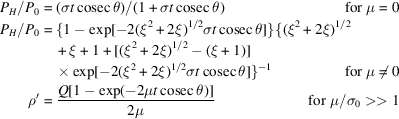2. Asymmetrical Bragg geometry, when the reflecting planes are inclined at an angleto the crystal surface, and the surface normal is in the plane of the incident and reflected beams.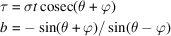angle of incidence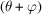and angle of emergence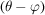to the crystal surface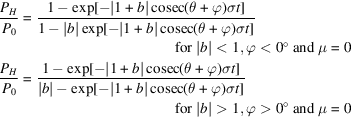Define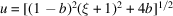and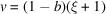: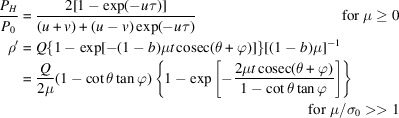(c) Transmission from a crystal slab of thickness t 1. Symmetrical Laue geometry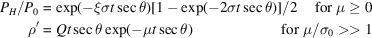2. Asymmetrical Laue geometry, when the reflecting planes are at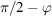to the crystal surface, with the normal in the plane of the incident and reflected beams.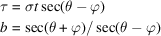angle of incidenceand angle of emergenceto the normal to the crystal surface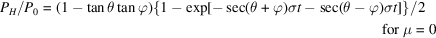Defineand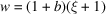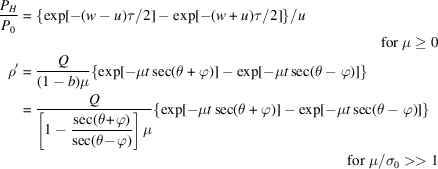(d) Powder halo: no absorption correction included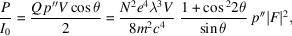where P is the diffracted power. (e) Debye–Scherrer lines on cylindrical film: no absorption correction included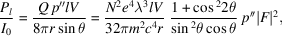where l is the length of line measured and r is the radius of the camera. Pl is the power reflected into length l. (f) Reflection from a thick block of powdered crystal of negligible transmission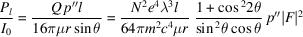(g) Transmission through block of powdered crystal of thickness t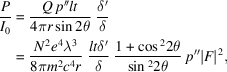where δ′, δ are the densities of the block of powder and of the crystal in bulk, respectively. (h) Rotation photograph of small crystal, volume V 1. Beam normal to axis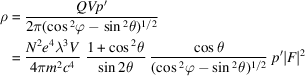2. Equi-inclination Weissenberg photograph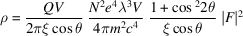Symbols
Q Integrated reflection from a crystal of unit volume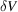Volume of crystal element
e, m Electronic charge and mass
c Speed of light
λ Wavelength of radiation
μ Linear absorption coefficient for X-rays or total attenuation coefficient for neutrons
Angle between incident and diffracted beamsIn (b) and (c), as defined; in (h), latitude of reciprocal-lattice point relative to axis of rotation
V Volume of crystal, or of irradiated part of powder sample
N Number of unit cells per unit volume
ξ In (b) and (c), as defined; in (h), radial coordinate xi used in interpreting Weissenberg photographs
I0 Energy of radiation falling normally on unit area per second
hkl Indices of reflection
F Structure factor of hkl reflection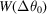Distribution function of the mosaic blocks at angular deviationfrom the average reflecting plane
σ Diffraction cross section per unit volume
σ0 Diffraction cross section per unit volume at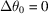b Asymmetry parameter
τ Reduced thickness of the crystal slab
PH/P0 Reflection power ratio, i.e. the ratio of the diffracted power to the incident power
ρ Integrated reflection power ratio from a crystal element
ρ′ Integrated reflection power ratio, angular integration of reflection power ratio
p Multiplicity factor for single-crystal methods
p′′ Multiplicity factor for powder methods

A conspectus of the expressions for the intensity of diffraction as recorded by various techniques, including the fundamental constants as well as the trigonometric factors, is given in Table 6.2.1.1. Details of the techniques are given elsewhere in this volume (Chapters 2.12.3) and in textbooks, such as those of Arndt & Willis (1966) for single-crystal diffractometry and Klug & Alexander (1974) for powder techniques. Notes on individual factors follow.

### References

Arndt, U. W. & Willis, B. T. M. (1966). Single crystal diffractometry. Cambridge University Press.
Kasper, J. S. & Lonsdale, K. (1959). International tables for X-ray crystallography. Vol. II. Mathematical tables. Birmingham: Kynoch Press.
Kasper, J. S. & Lonsdale, K. (1972). International tables for X-ray crystallography. Vol. II. Mathematical tables. Corrected reprint. Birmingham: Kynoch Press.
Klug, H. P. & Alexander, L. E. (1974). X-ray procedures for polycrystalline and amorphous materials. New York: John Wiley.